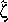# Analytic Number Theory and Applications: Collection of papers

To Prof. Anatolii Alexeevich Karatsuba, on occasion of his 60th birthday

Proc. V.A. Steklov Inst. Math. 218 (1997)

ISBN 5-02-003707-9

This is a collection of papers containing original results in additive number theory, Riemann's Zeta-function and its generalizations, Diophantine approximations, algebraic geometry, oscillating integrals, functions of a real variable, complexity of algorithms and schemes of functional elements.

The book is of interest to researchers and postgraduate students working in analytic number theory and its applications, algebraic geometry, mathematical cybernetics.

Contents (dvigzip ps)
G.I. Arkhipov and V.N. Chubarikov "On Professor A.A. Karatsuba's Works in Mathematics" (dvigzip ps) 1–14
V.B. Alexeev "From the Karatsuba Method for Fast Multiplication of Numbers to Fast Algorithms for Discrete Functions" (dvigzip ps) 15–22
G.I. Arkhipov, K. Buriev, and V.N. Chubarikov "On the Power of a Singular Set in Binary Additive Problems with Prime Numbers" (dvigzip ps) 23–52
V.I. Bernik and V.N. Borbat "Simultaneous Approximation of Zero by Values of Integral Polynomials" (dvigzip ps) 53–68
S.V. Bochkarev "On One Method of Estimation of the L1-Norm of an Exponential Sum" (dvigzip ps) 69–71
S.M. Voronin "On Interpolation Formulas" (dvigzip ps) 72–93
M.Z. Garaev "Third-Degree Diophantine Equations" (dvigzip ps) 94–103
R. Garunkshtis and A. Laurinchikas "Limit Theorem with Weight for the Lerch Zeta Function in the Space of Analytic Functions" (dvigzip ps) 104–116
S.B. Gashkov "On the Complexity of Integration of Rational Fractions" (dvigzip ps) 117–128
S.A. Gritsenko "On the Zeros of Linear Combinations of the Analogs of Riemann's Function" (dvigzip ps) 129–145
V.G. Zhuravlev "Orbits of Representation of Numbers by Local Quadratic Forms" (dvigzip ps) 146–159
V.V. Zudilin "Difference Equations and the Irrationality Measure of Numbers" (dvigzip ps) 160–174
I.A. Ikromov "On the Exponent of Convergence of Trigonometric Integrals" (dvigzip ps) 175–185
V.A. Iskovskikh "On the Rationality Problem for Three-Dimensional Algebraic Varieties" (dvigzip ps) 186–227
O.M. Kasim-Zadeh "The Complexity of Circuits of Unit Resistances and Some Properties of Fibonacci Numbers" (dvigzip ps) 228–242
L.A. Kogan "Representation of Numbers by Quadratic Forms" (dvigzip ps) 243–256
F.M. Malyshev "Optimisation Problem for the Brunn–Minkowski Inequality" (dvigzip ps) 257–260
D.A. Mit'kin "On the Lower Bound of the Number of Variables for the Solvability of the Hilbert–Kamke System in 3-Adic Integers" (dvigzip ps) 261–281
J. Moser "The Function–1(s) and Formulas of the Second Generation for π(x) and R(x)" (dvigzip ps) 282–293
Yu.V. Nesterenko "On the Measure of Algebraic Independence of the Values of Ramanujan Functions" (dvigzip ps) 294–331
V.I. Nechaev "Distribution of Signs in a Sequence of Rectangular Matrices over a Finite Field" (dvigzip ps) 332–339
A.I. Pavlov "Meromorphic Continuation of Dirichlet Series Connected with the Distribution of Numbers Modulo 1" (dvigzip ps) 340–351
D.A. Popov "Spherical Convergence of the Fourier Series and Integral of the Indicator of a Two-Dimensional Domain" (dvigzip ps) 352–371
V.I. Skalyga "Estimates of the Derivatives of Polynomials on Convex Bodies" (dvigzip ps) 372–383
H.M. Saliba "On the Mean Value of the Ternary Function of Divisors on a Sparse Sequence" (dvigzip ps) 384–395
N.T. Temirgaliev "On the Construction of Probability Measures on Functional Classes" (dvigzip ps) 396–401
N.M. Timofeev "Distribution in the Mean in Progressions of Numbers with a Large of Prime Factors" (dvigzip ps) 402–413
D.I. Tolev "On the Number of Representations of an Odd Integer as a Sum of Three Primes, One of Which Belongs to an Arithmetic Progression" (dvigzip ps) 414–432
V.G. Chirskii "On the Algebraic Independence of the Values of Functions Satisfying Systems of Functional Equations" (dvigzip ps) 433–438
A.B. Shidlovskii "On the Algebraic Independence of the Values of E-Functions" (dvigzip ps) 439–443
V.A. Yudin "On Trigonometric Series with Positive Partial Sums" (dvigzip ps) 444–447

Started on July 2, 1997